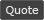WoWInterface - View Single Post - Nameplate Aura Filter
 Thread: Nameplate Aura Filter View Single Post
01-21-22, 02:50 AM   #7
Zaqqari
A Deviate Faerie Dragon
Join Date: Aug 2019
Posts: 11
Hey, thanks for the help! It's not working at all. It defaults back to Blizzards nameplate auras. Here is the error report from BugGrabber:

Lua Code:
`21x ...eNameplateAuraFilter\NameplateAuraFilter-1.0.lua:1797: attempt to index field 'UnitFrame' (a nil value)[string "@NameplateAuraFilter\NameplateAuraFilter-1.0.lua"]:1797: in function <...eNameplateAuraFilter\NameplateAuraFilter.lua:1796>[string "@NameplateAuraFilter\NameplateAuraFilter-1.0.lua"]:1821: in function <...eNameplateAuraFilter\NameplateAuraFilter.lua:1821> Locals:nameplate = NamePlate1 { OnSizeChanged = <function> defined @Blizzard_NamePlates\Blizzard_NamePlates.lua:485 GetPreferredInsets = <function> defined @Blizzard_NamePlates\Blizzard_NamePlates.lua:466 GetAdditionalInsetPadding = <function> defined @Blizzard_NamePlates\Blizzard_NamePlates.lua:455 ApplyOffsets = <function> defined @Blizzard_NamePlates\Blizzard_NamePlates.lua:437 OnRemoved = <function> defined =[C]:-1 OnAdded = <function> defined =[C]:-1 template = "NamePlateUnitFrameTemplate" 0 = <userdata> OnOptionsUpdated = <function> defined @Blizzard_NamePlates\Blizzard_NamePlates.lua:431}(*temporary) = nil(*temporary) = nil(*temporary) = "attempt to index field 'UnitFrame' (a nil value)"whitelist = <table> { 358259 = "all" 22703 = "all" 25771 = "all" 201158 = "all" 45334 = "all" 202274 = "all" 453 = "all" 221527 = "all" 31935 = "all" 208618 = "all" 206961 = "all" 200108 = "all" 211015 = "all" 317589 = "all" 202244 = "all" 331866 = "player" 324748 = "player" 212540 = "all" 118 = "all" 202914 = "all" 323996 = "all" 91800 = "all" 119611 = "player" 217832 = "all" 205369 = "all" 119381 = "all" 15487 = "all" 324263 = "all" 221562 = "all" 122783 = "player" 324867 = "player" 323524 = "player" 307871 = "all" 205147 = "player" 20066 = "all" 125174 = "player" 212638 = "all" 325886 = "all" 344021 = "player" 287254 = "all" 337341 = "player" 196733 = "player" 337571 = "player" 10326 = "all" 356567 = "all" 213691 = "all" 116680 = "player" 207736 = "all" 236273 = "all" 2094 = "all" 61305 = "all" 161353 = "all" 236077 = "all" 233582 = "all" 343294 = "all" 115804 = "player" 123725 = "player" 202090 = "player" 325190 = "player" 161354 = "all" 199085 = "all" 196608 = "player" 199845 = "all" 31661 = "all" 206649 = "all" 202346 = "all" 290512 = "player" 117526 = "all" 5211 = "all" 215479 = "player" 198819 = "all" 132169 = "all" 205630 = "all" 196737 = "player" 33395 = "all" 334693 = "all" 5246 = "all" 99 = "all" 213688 = "all" 408 = "all" 305485 = "all" 247483 = "player" 243435 = "player" 195630 = "player" 209584 = "player" 89766 = "all" 287712 = "all" 233395 = "all" 203337 = "all" 87204 = "all" 20549 = "all" 6789 = "all" 6358 = "all" 5484 = "all" 1098 = "all" 24394 = "all" 126819 = "all" 6770 = "all" 171017 = "all" 322459 = "all" 211881 = "all" 30283 = "all" 61721 = "all" 853 = "all" 196942 = "all" 196741 = "player" 325202 = "player" 269352 = "all" 28271 = "all" 122278 = "player" 323673 = "all" 211010 = "all" 1513 = "all" 137639 = "player" 353509 = "player" 2637 = "all" 132578 = "player" 1833 = "all" 19386 = "all" 353319 = "all" 197908 = "player" 116095 = "player" 196725 = "player" 202162 = "player" 204399 = "all" 9484 = "all" 197214 = "all" 123586 = "player" 207167 = "all" 209749 = "all" 277778 = "all" 353503 = "player" 204490 = "all" 91807 = "all" 118345 = "all" 77505 = "all" 64695 = "all" 82691 = "all" 212332 = "all" 51514 = "all" 191840 = "player" 324382 = "all" 162480 = "all" 248646 = "player" 1776 = "all" 1330 = "all" 212150 = "all" 207777 = "all" 345230 = "player" 196364 = "all" 41425 = "all" 132168 = "all" 200196 = "all" 199042 = "all" 277784 = "all" 102359 = "all" 64044 = "all" 205290 = "all" 179057 = "all" 605 = "all" 207171 = "all" 203123 = "all" 325216 = "player" 233022 = "all" 202335 = "player" 325153 = "player" 277787 = "all" 204080 = "all" 116847 = "player" 115078 = "all" 255941 = "all" 202933 = "all" 28272 = "all" 217824 = "all" 8122 = "all" 332423 = "all" 325092 = "player" 105771 = "all" 212337 = "all" 116768 = "player" 198121 = "all" 336887 = "player" 163505 = "all" 201787 = "player" 228287 = "player" 108194 = "all" 277792 = "all" 105421 = "all" 198909 = "all" 124280 = "player" 122470 = "player" 228563 = "player" 207685 = "all" 121253 = "player" 233759 = "all" 107079 = "all" 210873 = "all" 116706 = "all" 116189 = "player" 354540`

Last edited by Zaqqari : 01-21-22 at 03:07 AM.